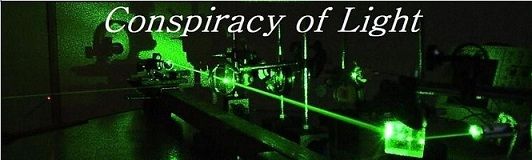The search for  new physics.

Explanation for the lack of terrestrial aberration in a Michelson Interferometer:

Doug Marett (2013)

Introduction:

Although it is now widely accepted that a moving Michelson interferometer will experience no fringe shifts due to its velocity, a simple analysis of the moving mirrors with respect to the laser light does suggest that the beams should undergo some slight terrestrial aberration. For example, Janssen  pointed out that the assumptions of Lorentz ether theory when applied to the moving interferometer might lead to the mirrors not reflecting light according to the standard laws of reflection.  Sir Oliver Lodge argued in favour of Lorentz’s explanation, by referring to the effect of the Fitzgerald-Lorentz contraction on the apparatus in preserving the angles and optical path lengths. This issue was addressed in detail by Herbert Ives in 1937, who argued that “The Fitzgerald-Lorentz contraction may be considered a means of introducing a tilt of the inclined mirror just sufficient to correct this error and bring the two emergent pulses into coincidence.” If the Lorentz contraction is not considered, then an “error of reflection” on the order of ½ v^2/c^2 arises, a term Ives attributes to Lodge. I would like to discuss herein the arguments used by Ives to explain why no second order terrestrial aberration arises in the moving Michelson interferometer.

Explanation:

Let’s consider a simple Michelson interferometer as shown in Fig. 1:

Fig. 1: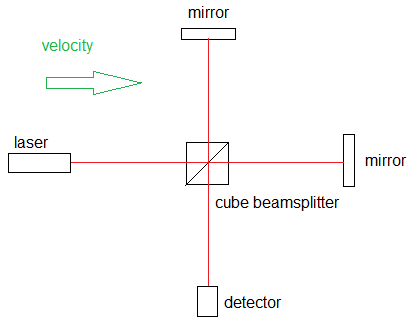The velocity is left to right. Let’s consider the situation where the velocity is at ½ the speed of light, i.e.   v = 0.5C:

From the time of emission to the time that the light reaches the beam splitter and mirrors, the optical components have moved to the right at 0.5C The beam splitter mirror at 45 degrees does not reflect the beam perpendicular as shown but must reflect the beam at a forward angle to meet the upper mirror at its new position. The ultimate reflection is at a virtual 60 degrees from vertical rather than 45 due to the composition of velocities, using the law of Sines. This is shown below in Fig 2.

Fig. 2: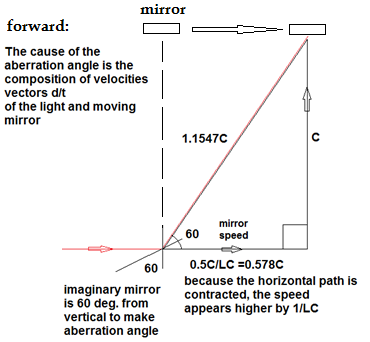In the case of the return beam, the light is reflected at more of an angle backwards due to the forward motion of the beamsplitter mirror. In the return case the virtual mirror appears as if it is at an angle of 30 degrees to the vertical due to aberration as shown in Fig. 3.

Fig. 3: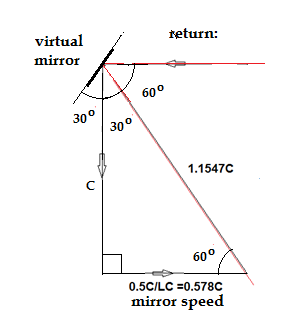Putting it all together, the interferometer stretched in velocity is shown below in Fig. 4:

Fig. 4: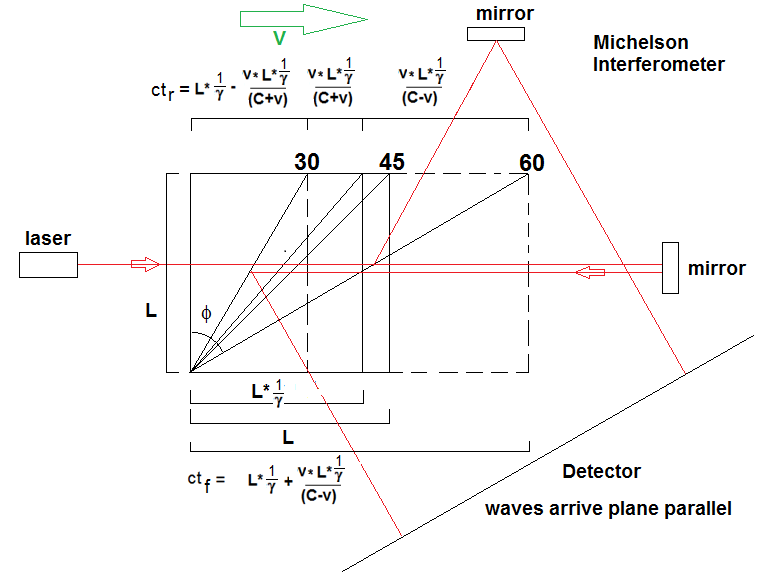As is shown in Fig. 4, the Lorentz contraction, denoted as 1/g, is included in the calculation both for the contraction of the length of the parallel path due to the motion, as well as the tilt of the beamsplitter due to the contraction. Both of these effects add to the aberration angle to insure that the return beams arrive at the detector perfectly parallel. If the Lorentz contraction is neglected, then a significant angle error will appear that is obvious at high velocities. The necessity of requiring a physical Lorentz contraction to make the beams arrive at the detector straight (as occurs in reality) suggests that the original idea of Fitzgerald, Lorentz and Larmor about the contraction of moving matter is valid and not just an ad hoc hypothesis. This idea was verified by Herbert Ives in his paper “A graphical Exposition of the Michelson Morley Experiment.” (1937).

Using Ives calculation method:

I have created a simulator to demonstrate this effect, click here to open the simulator. This uses a modification of Herbert Ives method to calculate the virtual angle of the moving beam splitter mirror to the vertical, and thereby the angles at which the beams arrive at the detector. The expected angle f for the beamsplitter mirror seen by either the forward or return beam is worked out by using the Law of Sines, knowing the virtual lengths of the stretch or contracted beamsplitter cube based on the values L and ctf   or  ctr as shown in fig. 4.

angle f = ACOS (L/SQRT(ct^2+L^2))

For the forward condition (Lorentz contraction included, yes/no = 0):

Use ctf in equation.    angle f = 60 degrees when 1/g = 0.866  (equation is shown in Fig.4)

For the reverse condition (Lorentz contraction included, yes/no = 0):

Use ctr in equation.   angle f = 30 degrees when 1/g = 0.866 (equation is shown in Fig. 4)

In viewing the above diagram, 60 and 30 degrees respectively results in the two beams reaching the detector plane parallel when the Lorentz contraction of the beamsplitter and path lengths are accounted for.

In the simulator, the Lorentz contraction can be added or removed to see the difference in the result. If 1/g is set to yes/no =1 in the simulator instead (no Lorentz contraction) then:

ctf = 63.435 degrees

Ctr = 33.690 degrees

Thus without the Lorentz contraction of both the length and the resultant twist in the mirror angle, the beams will not return rectilinearly to the detector in the Michelson interferometer. The angles should diverge from each other by 7.125 degrees.

Discussion and Conclusions

This exercise hopefully demonstrates that not only is a physical Lorentz contraction necessary to explain the lack of fringe shift, it is also necessary to explain the lack of beam divergence at the detector due to our motion though space. This issue was also addressed in detail by Gjurchinovski (2004) in a detailed analysis of the reflection of light from moving mirrors. He states:

“A common interpretation of the effect of Lorentz contraction is that relativistic contraction is not a real, physical contraction, but an apparent, artiﬁcial phenomenon, whose presence is solely due to the process of relativistic measurement of the length…however, we showed that Lorentz contraction is a real effect and is an inherent physical property of a mirror (and, therefore, of any object) in uniform rectilinear motion. As a physical property of an object in motion, Lorentz contraction is not just a result of the process of relativistic measurement of the object’s length.” 

In this regard, Gjurchinovski  appears to concur with Ives that the Fitzgerald-Lorentz contraction is a real, physical contraction of the optical apparatus, and that the relativistic interpretation of the Lorentz contraction does not fit the data.

References:

 Sir Oliver Lodge, The Ether of Space (1909) Harper Brothers, P. 140.

 Herbert Ives, “Graphical Exposition of the Michelson Morley Experiment”. Optical Soc. Am. Vo. 27 No. 5 1937. P.177 – 180.

 Janssen, Michael, http://www.mpiwg-berlin.mpg.de/litserv/diss/janssen_diss/Chapter3.pdf

 Aleksandar Gjurchinovski, “Reﬂection of light from a uniformly moving mirror. Am. J. Phys. 72 (10), October 2004.